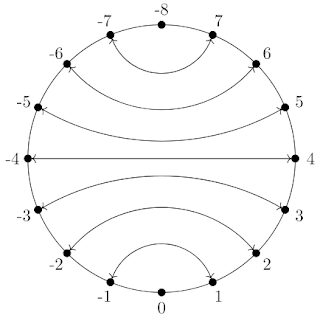## Sunday 6 August 2017

### Visualizing addition, subtraction and bitwise complement

A relatively well-known relation between addition and subtraction, besides the basic relations a - b = a + (-b) and a - b = -(-a + b), is a - b = ~(~a + b). But I suspect most people have simply accepted that as fact, or perhaps proved it from the 2's complement definition of negation. haroldbot can do the latter, though not as succinctly as I hoped.

But it also has a nice one-dimensional geometric interpretation, really analogous to the way a - b = -(-a + b) looks in ℤ.

As negation mirrors ℤ around the origin, complement mirrors the space of two's complement signed bitvectors around the "space" between -1 and 0. Clearly addition in the mirrored space corresponds to subtraction in the unmirrored space, so the obvious way to subtract is mirror, add, and mirror back. That's precisely what -(-a + b) does in ℤ and what ~(~a + b) does for bitvectors. An observant reader may notice that I convenient disregarded the finiteness of the number line of fixed-size bitvectors, that's actually not a problem but the visualization gets a bit trickier.

What is in a way more surprising is that a - b = -(-a + b) works for bitvectors, since negation does not neatly mirror the whole number line when you're talking about two's complement negation. It's around the origin again instead of around a "space", but the most negative number is unaffected.

When we remember that this number line is really a number ring (in the circular sense), that starts to make sense again. To complete this picture, you can think about holding a ring in your hands, flipping it over while holding it at two diametrically opposite points - zero and the most negative number. Of course this visualization also works for complement, just hold the ring at slightly different places: between negative one and zero, and between the minimum and maximum (which are adjacent, you could think of it as where the ring closes). There are images below, but you should probably only look at them if visualization failed to appear in your mind naturally - if you already have one, your own image is probably easier to think about.

### But why

OK all this spatial insight is fun (maybe), but what was it actually good for. I've found that thinking about the complement operation this way helps me to relate it to addition-like arithmetic operations (add, subtract, min, max, compare, etc) since they're all simple operations with "arrows" around that ring that we just flipped in our minds.

So it helps to make sense of various "mutants" of De Morgan's Law, such as:

• ~x < ~y == x > y
• ~x > ~y == x < y
• ~min(~x, ~y) == max(x, y)
• ~max(~x, ~y) == min(x, y)
• ~avg_up(~x, ~y) == avg_down(x, y) where avg_down is the average rounding down, see also VirtualDub: Weighted averaging in SSE (part 2)
• ~avg_down(~x, ~y) == avg_up(x, y)
• ~paddsb(~x, y) == psubsb(x, y) (signed saturation)
• ~psubsb(~x, y) == paddsb(x, y) (signed saturation)
• ~paddusb(~x, y) == psubusb(x, y) (unsigned saturation)
• ~psubusb(~x, y) == paddusb(x, y) (unsigned saturation)

A similar visualization works for the signed/unsigned "conversion" x ^ msb == x + msb == x - msb (msb is a mask with only the most significant bit set), which corresponds to rotating the ring 180 degrees. This may help when thinking about things such as:

• x <s y == (x ^ msb) <u (y ^ msb)
• x <u y == (x ^ msb) <s (y ^ msb)
• max_s(x, y) == max_u(x ^ msb, y ^ msb) ^ msb

The relationship between the different kinds of min/max can be summed up by a nice commutative diagram:

Hope it helps, for me this sort of thing has come in handy occasionally when writing SSE code.

Here are the images two's complement negation:and for plain one's complement:This is in the orientation that I usually use when I think about these operations this way, but there is not particular meaning to going counter-clockwise with 0 at/near the bottom.